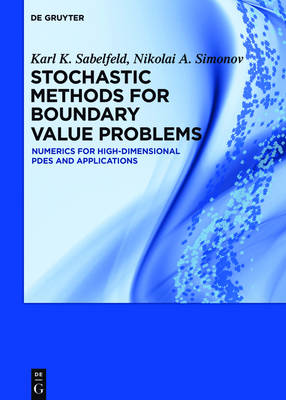Find your perfect holiday reading•# Stochastic Methods for Boundary Value Problems: Numerics for High-dimensional PDEs and Applications (Hardback)

(author), (author)
£91.00
Hardback 208 Pages / Published: 26/09/2016
• We can order this

Usually dispatched within 3 weeks

• This item has been added to your basket
This monograph is devoted to random walk based stochastic algorithms for solving high-dimensional boundary value problems of mathematical physics and chemistry. It includes Monte Carlo methods where the random walks live not only on the boundary, but also inside the domain. A variety of examples from capacitance calculations to electron dynamics in semiconductors are discussed to illustrate the viability of the approach. The book is written for mathematicians who work in the field of partial differential and integral equations, physicists and engineers dealing with computational methods and applied probability, for students and postgraduates studying mathematical physics and numerical mathematics. Contents: Introduction Random walk algorithms for solving integral equations Random walk-on-boundary algorithms for the Laplace equation Walk-on-boundary algorithms for the heat equation Spatial problems of elasticity Variants of the random walk on boundary for solving stationary potential problems Splitting and survival probabilities in random walk methods and applications A random WOS-based KMC method for electron-hole recombinations Monte Carlo methods for computing macromolecules properties and solving related problems Bibliography

Publisher: De Gruyter
ISBN: 9783110479065
Number of pages: 208
Weight: 525 g
Dimensions: 240 x 170 mm

MEDIA REVIEWS

"This book will be of great interest to any researcher who works in the eld of numerical solution of PDEs and integral equations."
Nikolaos Halidias in: Mathematical Review Clippings MR3676590 (2018)

## Reviews

Sign In To Write A Review

Please sign in to write a review

Your review has been submitted successfully.